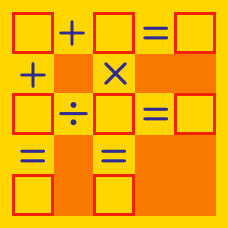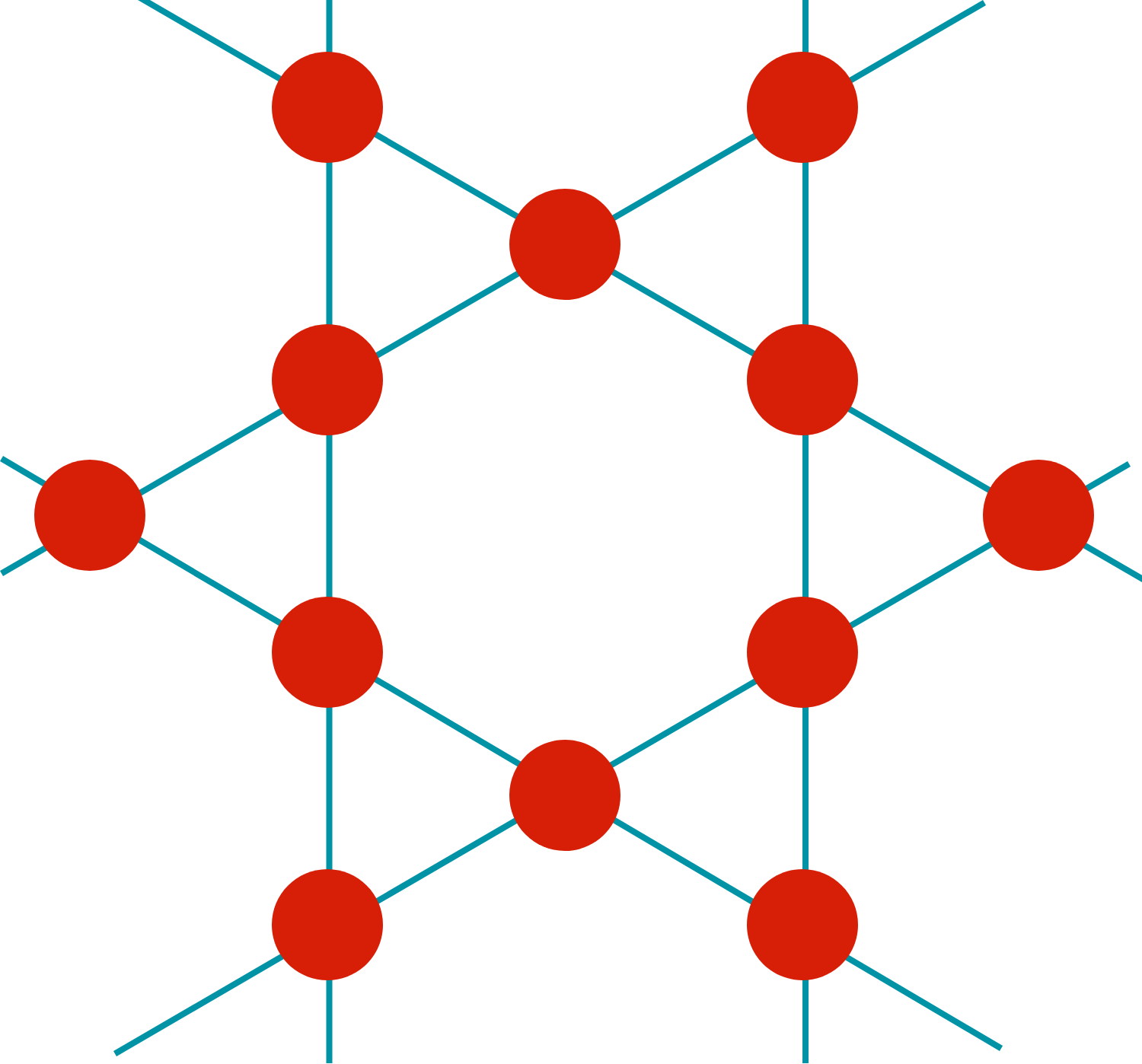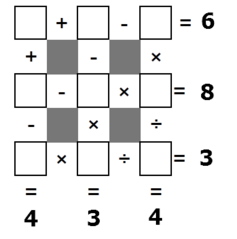Logic

Arithmetic Puzzles: Level 3 Challenges

$\large \left( \square \ + \ \square \ - \ \square \ \right) \times \ \square \ \div \ \square$

You are given that the numbers $1,2,3,4$ and $5$ are to be filled in the square boxes as shown above (without repetition).

Find the minimum possible value of the resultant number. Give your answer to 3 decimal places.

A distinct integer from 1 to 12 is placed in each red circle in the figure below. Is it possible that the sum of the four numbers on any of the six blue lines is the same?$\large \square + \square\square+\square\square\square+\square\square\square\square$

You are given that the numbers $0,1,2,\ldots,9$ are to be filled in the square boxes as shown above (without repetition) such that it represent a sum of a 1-digit, 2-digit, 3-digit, and 4-digit number.

Find total number of possible arrangements of these ten numbers such that the sum of these four numbers is maximized.

Details and Assumptions:

• For the purposes of this question, 0 is considered a 1-digit number.
• This is an arithmetic puzzle, where $1 \square$ would represent the 2-digit number 19 if $\square = 9$. It does not represent the algebraic expression $1 \times \square$.Using the digits 1 through 9 without repetition, fill out the number grid above. What is the product of the numbers in the 4 corners?

Note: The order in which this grid calculates is left-to-right/top-to-bottom unlike the usual order of operations. E.g., $1+2\times 3 = (1+2)\times3=9.$

$\large 1 \, \square \, 2 \, \square \, 3 \, \square \, 4 \, \square \, 5 \, \square \, 6 \, \square \, 7 \, \square \, 8 = 9$

There are $2^7 =128$ ways in which we can fill the squares with $+, -$.

How many ways would make the equation true?

Note: You are not allowed to use parenthesis.

×